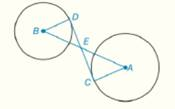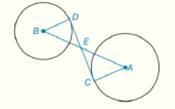Chapter 6.CR, Problem 27CRElementary Geometry For College St...

7th Edition
Alexander + 2 others
ISBN: 9781337614085

Solutions

Chapter
SectionElementary Geometry For College St...

7th Edition
Alexander + 2 others
ISBN: 9781337614085
Textbook Problem

In Review Exercises 27 to 29, give a proof for each statement. G i v e n :                     D C -   i s   t a n g e n t   t o   c i r c l e s   B   a n d   A   a t   p o i n t s   D   a n d   C ,   r e s p e c t i v e l y             P r o v e :                   A C · E D = C E · B DTo determine

To Prove: AC·ED=CE·BD, if DC- is tangent to circles B and A at points D and C, respectively.Explanation

Concept:

Theorem 6.2.3: The radius (or any other line through the center of a circle) drawn to a tangent at the point of tangency is perpendicular to the tangent at that point.

Calculation:

Given that DC- is tangent to circles B and A at points D and C, respectively.

By theorem 6.2.3, BDDE and ACCE.

So, BDE=ACE=90°

Because vertically opposite angles are equal, BED=AEC

Still sussing out bartleby?

Check out a sample textbook solution.

See a sample solution

The Solution to Your Study Problems

Bartleby provides explanations to thousands of textbook problems written by our experts, many with advanced degrees!

Get Started

7. Find the future value if \$8000 is invested for 10 years at compounded annually.

Mathematical Applications for the Management, Life, and Social Sciences

Find the median for the following set of scores: 1, 9, 3, 6, 4, 3, 11, 10

Essentials of Statistics for The Behavioral Sciences (MindTap Course List)

True or False: y = xex is a solution to y=y+yx.

Study Guide for Stewart's Single Variable Calculus: Early Transcendentals, 8th

2 1 0 does not exist

Study Guide for Stewart's Multivariable Calculus, 8th